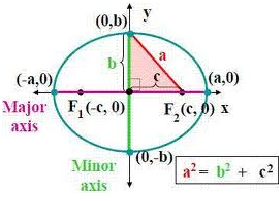×#### Thank you for registering.

One of our academic counsellors will contact you within 1 working day.

Click to Chat

1800-1023-196

+91-120-4616500

CART 0

• 0

MY CART (5)

Use Coupon: CART20 and get 20% off on all online Study Material

ITEM
DETAILS
MRP
DISCOUNT
FINAL PRICE
Total Price: Rs.

There are no items in this cart.
Continue Shopping• Complete JEE Main/Advanced Course and Test Series
• OFFERED PRICE: Rs. 15,900
• View Details

```Revision Notes on Ellipse

The standard equation of ellipse with reference to its principal axis along the coordinate axis is given by x2/a2 + y2/b2 = 1

In the standard equation, a > b and b2 = a2 (1 – e2) Hence, the relation between a and b is a2 – b2 = a2e2, where ‘e’ is the eccentricity and 0 < e < 1.The foci of the ellipse are S(ae, 0) and S’ = (-ae, 0)

Equations of the directrices are given by x = a/e and x = -a/e

The coordinates of vertices are A’ = (-a, 0) and A = (a,0)

The lengths of the major and minor axis are 2a and 2b respectively.

The length of latus rectum is 2b2/a = 2a(1 - e2)

Distance between the two foci is 2ae and distance between directrix is 2a/e.

Two ellipses are said to be similar if they have the same eccentricity.

The sum of the focal distances of any pint on the ellipse is equal to the major axis. As a result, the distance of focus from the extremity of a minor axis is equal to semi major axis.

The circle described on the major axis of an ellipse as diameter is called the auxiliary circle.

If a question does not mention the relation between a and b then by convention a is assumed to be greater than b i.e. a > b.

The point P(x1, y1) lies outside, inside or on the ellipse according as x12/a2 + y12/b2 – 1 >, < or = 0.

In parametric form, the equations x = a cos θ and y = b sin θ together represent the ellipse.

Comparison Chart between Standard Ellipse:?

Basic Elements

x2/a2 + y2/b2 = 1

a > b

a < b

centre

(0, 0)

(0, 0)

vertex

(±a, 0)

(0, ±b)

Length of major axis

2a

2b

Length of minor axis

2b

2a

foci

(± ae, 0)

(0, ± be)

Equation of directirx

x = ± a/e

y = ± b/e

Relation between a, b and c

b2 = a2(1 – e2)

a2 = b2(1 – e2)

Equation of major axis

y = 0

x = 0

Equation of minor axis

x = 0

y = 0

Length of latus rectum

2b2/a

2a2/b

Ends of latus rectum

(± ae, ± b2/a)

(± a2/b, ± be)

Distance between foci

2ae

2be

Distance between directrix

2a/e

2b/e

Parametric equation

(a cos θ, b sin θ) (0 < θ < 2π)

(a cos θ, b sin θ)

The line y = mx + c meets the ellipse x2/a2 + y2/b2 = 1 in either two real, coincident or imaginary points according to whether c2 is < = or > a2m2 + b2

The equation y = mx + c is a tangent to the ellipse if c2 = a2m2 + b2

The equation of the chord of ellipse that joins two points with eccentric angles α and β is given by
x/a cos (α + β)/2 + y/b sin (α + β)/2 = cos (α - β)/2

Equation of tangent to the ellipse:

The equation of tangent to the ellipse at the point (x1, y1) is given by xx1/a2 + yy1/b2 = 1

In parametric form, (x cos θ) /a + (y sin θ) /b = 1 is the tangent to the ellipse at the point (a cos θ, b sin θ).

Equation of normal:

Equation of normal at the point (x1,y1) is a2x/x1 – b2y/y1 = a2 - b2 = a2e2

Equation of normal at the point (a cos θ a, b sin θ) is ax sec θ – by cosec θ = (a2 - b2)

Equation of normal in terms of its slope ‘m’ is y = mx – [(a2 - b2)m /√a2 + b2m2]

The equation of director circle is x2 + y2 = a2 + b2

Chord of contact:

Pair of tangents drawn form outside point P(x1, y1) to the ellipse meet it at R and P. Line joining P and R is called the chord of contact of point P(x1, y1) with respect to the ellipse. The equation of chord of contact is

xx1/a2 + yy1/b2 = 1.The portion of the tangent to an ellipse between the point of contact and the directrix subtends a right angle at the corresponding focus.

The perpendiculars from the center upon all chords which join the ends of any particular diameters of the ellipse are of constant length.

Chord with a given middle point:

AB is a chord of the circle whose mid-point is P(x1, y1). Then the equation of the chord AB is T = S1, where

S1 = x12/a2 + y12/b2 – 1 and T = xx1/a2 + yy1/b2 – 1.

Two diameters of ellipse are said to be conjugate diameters if each bisects the chords parallel to the other.

The eccentric angles at the ends of a pair of conjugate diameters of an ellipse differ by a right angle.

The sum of squares of any two conjugate semi-diameters of an ellipse is constant and equal to the sum of squares of semi-axis of ellipse.

```### Course Features

• 728 Video Lectures
• Revision Notes
• Previous Year Papers
• Mind Map
• Study Planner
• NCERT Solutions
• Discussion Forum
• Test paper with Video Solution